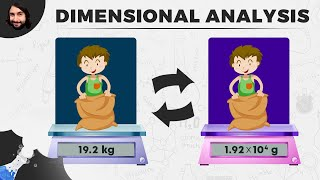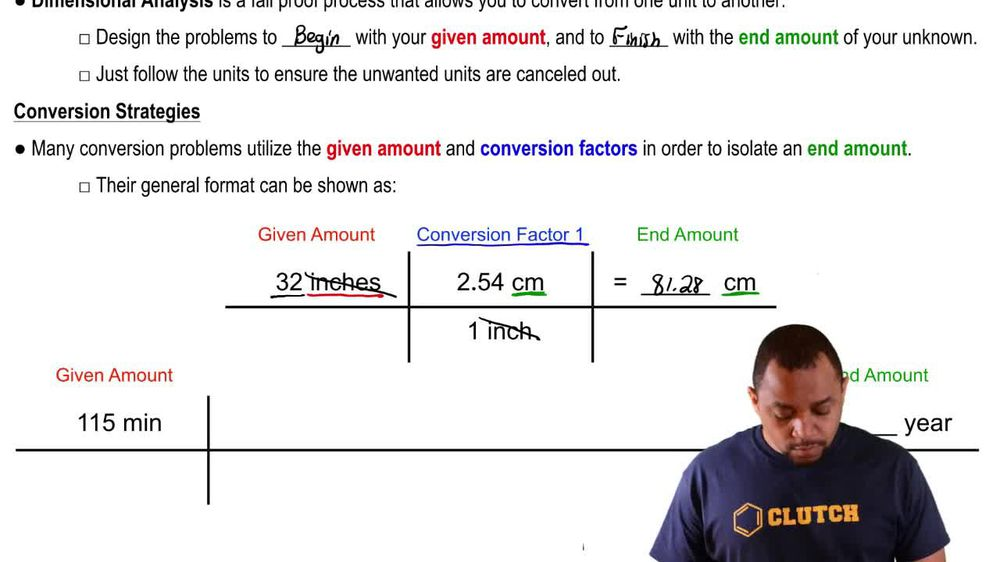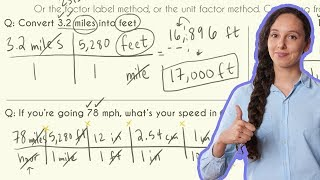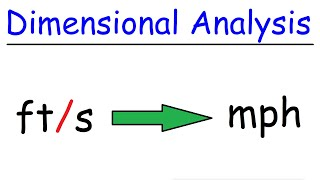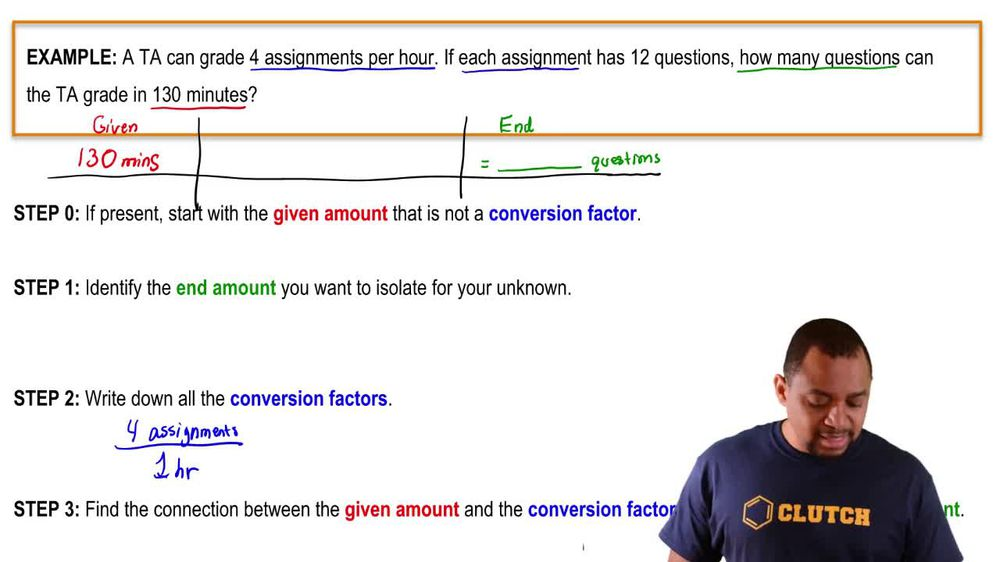Start typing, then use the up and down arrows to select an option from the list.
1. 1. Intro to General Chemistry2. Dimensional Analysis
Problem

# Carry out the following conversions. (c) How large in square meters is the land area of Australia (2,941,526 mi^2)?

Relevant Solution1m
Play a video:
Hey everyone, we're asked how large is the land area of Japan, 377,915 square kilometers in square miles. Taking our value of 377,915, km2 We're going to use the conversion factor that we've learned and this is going to be 1.6093 km is equivalent to one mile. But since we are in km2, we want to cancel out those units and we also want to convert our miles into square miles. So to do so, all we need to do is square both of these values. Now, when we calculate this out and cancel out our units, we end up with a value of 145, 921. sq mi and we can put this into scientific notation and this is going to be 1.4592 times 10 to the fifth square miles. And this is going to be our final answer. Now, I hope that made sense. And let us know if you have any questions